• 贝叶斯参数估计
2017-02-09 11:20:00

(学习这部分内容约需要1.9小时)

## 摘要

在贝叶斯框架中, 我们将统计模型的参数视为随机变量. 模型由变量值的先验分布以及决定参数如何影响观测数据的证据模型来指定. 当我们对观测数据进行条件化时, 我们得到参数的后验分布. 术语"贝叶斯参数估计"会让我们误以为对参数进行了估计, 实际上我们通常可以完全跳过参数估计步骤. 我们把参数积分掉, 并直接进行预测.

## 预备内容

弄清楚这个概念需要一些预备知识:

## 学习目标

• 知道"先验(prior)"和"似然函数(likelihood function)"是什么意思
• 可以使用贝叶斯公式(Bayes' Rule)计算后验分布
• 知道后验预测分布是什么, 对于简单的例子可以解析地计算后延预测分布(比如beta-Bernoulli模型)
• 什么是共轭先验(conjugate prior)? 为什么共轭先验有用?
• 为什么当使用共轭先验时, 后验分布可以根据pseudocounts给出?
• 什么是最大后验(maximum a-posteriori, MAP)近似? 给出一个MAP参数和后延预测分布不同的例子.

## 核心资源

(阅读/观看以下资源之一)

### 收费

• 概率图模型:原理和技术(Probabilistic Graphical Models: Principles and Techniques)
简介: 一本非常全面的关于概率AI的研究生课程的教科书
位置: Section 17.3, pgs. 733-741.
[网站]
作者: Daphne Koller, Nir Friedman
• Machine Learning: a Probabilistic Perspective(MLAPP)
简介: 一本非常全面的研究生机器学习教材
位置: Sections 3.1-3.3, pgs. 65-78
[网站]
作者: Kevin P. Murphy

## 增补资源

(以下为选修内容, 但你可能会发现它们很有用)

### 免费

• Coursera: Neural Networks for Machine Learning (2012)
简介: Geoff Hinton的在线课程, 他发明了许多神经网络和深度学习背后的核心理念
位置: "Introduction to the full Bayesian approach"
[网站]
作者: Geoffrey E. Hinton

### 付费

• 概率统计和数据分析(Mathematical Statistics and Data Analysis)
简介: 本科生统计教材
位置: Section 8.6, "The Bayesian approach to parameter estimation," up through 8.6.1, "Further remarks on priors," pages 285-296
[网站]
作者: John A. Rice
• 贝叶斯数据分析(Bayesian Data Analysis)
简介: 贝叶斯统计教科书, 着重于实际问题
位置: Sections 2.1-2.3, pgs. 33-39
[网站]
作者: Andrew Gelman,John B. Carlin,Hal S. Stern,Donald B. Rubin
• Pattern Recognition and Machine Learning(PRML)
研究生机器学习课程的教科书, 聚焦于贝叶斯方法
位置: Section 2.1, pgs. 68-74
[网站]
作者: Christopher M. BIshop

返回贝叶斯学习路线图

转载于:https://www.cnblogs.com/bayesianML/p/6381181.html

更多相关内容
• 参数估计 function [mu, sigma] = Bayesian_parameter_est(train_patterns, train_targets, sigma)
• HDDM是用于漂移扩散模型（通过PyMC）的分层贝叶斯参数估计的python工具箱。 漂移扩散模型被广泛用于心理学和认知神经科学中以研究决策。 查看有关如何入门的。 可以在下面以及部分和找到更多信息。 特征 使用DDM...
• 用于物理模型贝叶斯参数估计的迭代重要性采样 (IIS) 背景 该模块与有一定关系，但侧重于计算成本相对较高的物理模型。 它基于参数分布和结果模型状态的高斯近似，但在非线性偏差方面比集成卡尔曼滤波器更稳健 ( ) 在...Python
• 一、贝叶斯参数估计 前导篇：【机器学习】最大似然估计与最大后验估计 这里先回顾一下最大后验估计法： 最大后验估计法，通过最大化参数θθθ的后验分布来求出估计参数θ^\hat{θ}θ^ :θ^=argmax⁡θ⁡P(θ∣...

# 一、贝叶斯参数估计

• 这里先回顾一下最大后验估计法

• 最大后验估计法，通过最大化参数 θ θ 的后验分布来求出估计参数 θ ^ \hat{θ} : θ ^ = a r g max ⁡ θ ⁡ P ( θ ∣ x ) = a r g max ⁡ θ ⁡ P ( x ∣ θ ) ∗ P ( θ ) \hat{θ}=arg\max_θ⁡P(θ|x)=arg\max_θ⁡P(x|θ)*P(θ)
• 现在我们分析分析后验分布 P ( θ ∣ x ) P(θ|x) P ( θ ∣ x ) = P ( x ∣ θ ) ∗ P ( θ ) P ( x ) P(θ|x)=\frac{P(x|θ)*P(θ)}{P(x)} P ( x ) P(x) 为常数，对优化问题不影响，所以可以记作： P ( θ ∣ x ) = P ( x ∣ θ ) ∗ P ( θ ) P(θ|x)=P(x|θ)*P(θ)
• P ( x ∣ θ ) P(x|θ) 为样本的概率分布， P ( θ ) P(θ) 为参数 θ θ 的先验分布。我们将样本 x x 带入后： P ( θ ∣ x ) P(θ|x) 就是一个 θ θ 的概率分布，即 θ θ 后验分布。既然它是一个概率分布，我们可以简单的把它理解为一个概率密度函数 f ( θ ) f(θ) ，即 f ( θ ) = P ( θ ∣ x ) f(θ)=P(θ|x) ，最大后验估计法就是用该函数 ( f ( θ ) ) (f(θ)) 的最大值点作为我们估计的参数: θ ^ \hat{θ}

• 贝叶斯参数估计法是最大后验估计法的加强版

• 先引入损失函数 L ( θ , a ) L(θ,a) ,这个地方对于损失函数中变量的含义，很多博客和资料说法都不一致，这里只是根据个人理解给出中间两个变量的含义：
• θ θ ：是后验分布的随机变量， θ θ 的分布函数为： f ( θ ) = P ( θ ∣ x ) f(θ)=P(θ|x)
• a a ：也是一个随机变量，也可以理解为一个普通自变量，最后用 a a 来估计贝叶斯参数估计法得出的 θ ^ \hat{θ}
• 参数的后分布 P ( θ ∣ x ) P(θ|x) 还有一个含义:原本参数 θ θ 是服从先验分布 P ( θ ) P(θ) 的，当我们获得样本数据 x x 后，将样本数据的以下统计信息加入到 θ θ 分布中后， θ θ 变成服从后验验分布 P ( θ ∣ x ) P(θ|x) 的随机变量了。
• 现在假设我们有了样本数据 X X ，和损失函数 L ( θ , a ) L(θ,a)
• 首先我们将样本信息加入到 θ θ 分布中，得到 θ θ 当前服从的后验分布 P ( θ │ x ) P(θ│x)
• 得到了参数 θ θ 当前的概率分布 P ( θ │ x ) P(θ│x) 后，我们就可以计算损失函数 L ( θ , a ) L(θ,a) 相对参数 θ θ 的数学期望了： E θ ( L ( θ , a ) ) = ∫ L ( θ , a ) P ( θ │ x ) d θ E_θ (L(θ,a))=∫L(θ,a)P(θ│x)dθ 这是一个a的函数叫做：贝叶斯风险
• 最后贝叶斯参数估计法通过最小化贝叶斯风险得到最后的参数估计值 θ ^ \hat{θ} ，即： θ ^ = a r g min ⁡ a ⁡ E θ ( L ( θ , a ) ) \hat{θ}=arg\min_a⁡E_θ (L(θ,a))

• 贝叶斯参数估计法步骤：

• 第一步：计算后验分布 P ( θ │ x ) P(θ│x) P ( θ │ x ) = P ( x │ θ ) ∗ P ( θ ) P ( x ) P(θ│x)=\frac{P(x│θ)*P(θ)}{P(x)}
• 第二步：计算贝叶斯风险： E θ ( L ( θ , a ) ) = ∫ L ( θ , a ) P ( θ ∣ x ) d θ E_θ (L(θ,a))=∫L(θ,a) P(θ|x)dθ
• 第三步：最小化贝叶斯风险得到 θ ^ \hat{θ} θ ^ = a r g min ⁡ a ⁡ E θ ( L ( θ , a ) ) \hat{θ}=arg \min_a⁡E_θ (L(θ,a))

• 由上面的推导过程我们知道，贝叶斯参数估计法求解过程有两个难点

• 第一个：求后验分布
• 第二步：最小化贝叶斯风险，涉及积分以及最小值求解

# 二、共轭分布

• 共轭先验:设资料 X X 有概率密度函数 F ： X ∼ F ( x ∣ θ ) F：X∼F(x|θ) . θ θ 的先验分布 π ( θ ) π(θ) 属于某个分布族 P ： π ( θ ) ∈ P . P：π(θ)∈P. 如果 θ θ 的后验分布: π ( θ ∣ x ) π(θ|x) 也属于分布族 P P ，那么 P P 就叫做 F F 的共轭先验。
• 假设样本 x x 的分布为： f ( x ∣ θ ) f(x|θ) θ θ 的先验分布 π ( θ ) π(θ) 分布已知。如果 π ( θ ) π(θ) f ( x ∣ θ ) f(x|θ) 共轭，那么 π ( θ ) π(θ) π ( θ ∣ x ) π(θ|x) 会有一样的形式，这样不就会很方便求解后验分布 π ( θ ∣ x ) π(θ|x) 了吗？这样将先验分布设计成与 f ( x ∣ θ ) f(x|θ) 共轭，后面计算会很方便。
• 幸运的是我们可以证明，所有属于指数族分布 f ( x ∣ θ ) f(x|θ) ，都可以求出它的共轭先验分布 π ( θ ) π(θ) 的具体形式。而大部分常见的分布都属于指数族分布，比如正态分布，指数分布，二项分布，泊松分布，Beta分布，Gamma分布等等。
• 常用的共轭分布：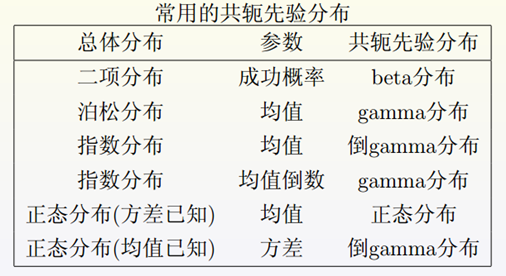其中整体分布，就是样本分布 f ( x ∣ θ ) f(x|θ) 。如果你的样本的分布是上面表中第一列中的一项，那么就可以将先验分布设计成第三列中对应的分布。所以在才会在那么多算法或实际问题中将某个参数的先验分布定义成Gamma或Beta这种“奇怪”的形式，原因就是为了求解方便。这就解决了第一个难题：求后验分布

## Gamma 分布：

• Gamma函数： Γ ( x ) = ∫ 0 ∞ t x − 1 e − t d t Γ(x)=∫_0^∞t^{x-1} e^{-t} dt
• Gamma函数图像：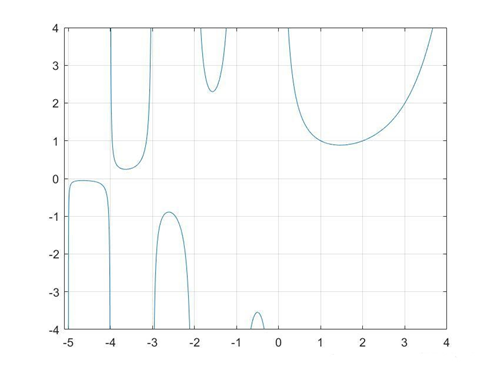• 通过分部积分法，可以很容易证明Gamma函数具有如下之递归性质： Γ ( x + 1 ) = x Γ ( x ) Γ(x+1)=xΓ(x) Γ ( x ) = ( x − 1 ) ! Γ(x)=(x-1)!
• Gamma分布:
• 根据 Γ Γ 函数的定义有： ∫ 0 ∞ x α − 1 e − x Γ ( α ) d x = 1 ∫_0^∞\frac{x^{α-1} e^{-x}}{Γ(α)} dx=1
• 取积分中的函数作为概率密度，就得到了一个形式最简单的Gamma分布，其概率密度函数为： G a m m a ( x ∣ α ) = x α − 1 e − x Γ ( α ) Gamma(x|α)=\frac{x^{α-1} e^{-x}}{Γ(α)} 其中, α α 为Gamma分布的shape parameter，主要决定了曲线的形状；而 β β 为Gamma分布的 rate parameter，主要决定了曲线有多陡。Gamma分布的归一化常数恰为 Γ Γ 函数在点 α α 处的值 Γ ( α ) Γ(α)
• Gamma分布的期望、方差： E ( t ) = α β E(t)=\frac{α}{β} D ( t ) = α β 2 D(t)=\frac{α}{β^2}
• Gamma分布的图像：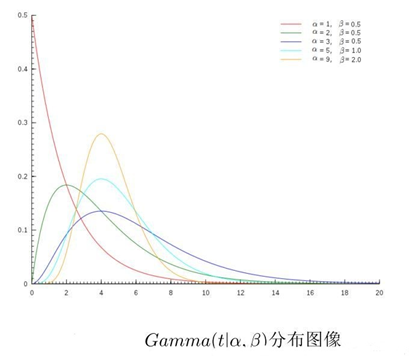## Beta分布：

• Beta函数： B ( α , β ) = ∫ 0 1 x α − 1 ( 1 − x ) β − 1 d x B(α,β)=∫_0^1x^{α-1} (1-x)^{β-1} dx 其中 α , β &gt; 0 α,β&gt;0
• Beta函数性质:
• 对称性: B ( α , β ) = B ( β , α ) B(α,β)=B(β,α)
• Γ Γ 函数的关系： B ( α , β ) = Γ ( α ) Γ ( β ) Γ ( α + β ) B(α,β)=\frac{Γ(α)Γ(β)}{Γ(α+β)}
• 根据Beta函数的定义有： ∫ 0 1 p α − 1 ( 1 − p ) β − 1 B ( α , β ) d p = 1 ∫_0^1\frac{p^{α-1} (1-p)^{β-1}}{B(α,β)} dp=1
• 上式中取积分中的函数作为概率密度，就得到了Beta分布： B ( p ∣ α , β ) = p α − 1 ( 1 − p ) β − 1 B ( α , β ) B(p|α,β)=\frac{p^{α-1} (1-p)^{β-1}}{B(α,β)}
• 可以发现Beta分布的归一化常数恰为Beta函数在 ( α , β ) (α,β) 处的值.
• Beta 分布的期望、方差： E ( p ) = α β + α E(p)=\frac{α}{β+α} D ( p ) = β α ( β + α ) 2 ( β + α + 1 ) D(p)=\frac{βα}{(β+α)^2 (β+α+1)}
• Beta 分布的图像：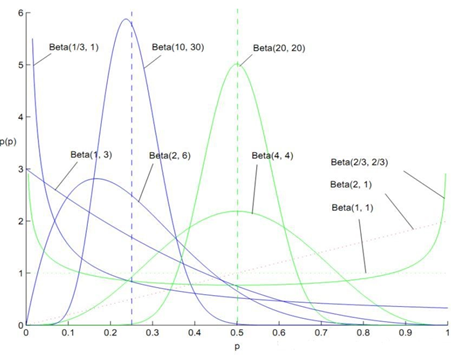# 三、损失函数

• 贝叶斯估计的最后一步需要求下面式子： θ ^ = a r g min ⁡ a ⁡ E θ ( L ( θ , a ) ) \hat{θ}=arg\min_a⁡E_θ (L(θ,a)) 其中涉及积分以及最小值求解，看起来十分麻烦。所幸的是在特定的损失函数形式下，上面最后一步可以化简，并不需要完全用到积分。下面一部分的讲解就是在三种特定损失函数形式下贝叶斯估计的计算方法。
• 平方损失函数：
• 公式： L ( θ , a ) = ( θ − a ) 2 L(θ,a)=(θ-a)^2
• 若损失函数是平方损失函数，那么 θ ^ \hat{θ} 等于后验概率 π ( θ ∣ x ) π(θ|x) 期望值，即当 a a 等于 θ θ π ( θ ∣ x ) π(θ|x) 上的期望时，贝叶斯风险最小。
• 绝对值损失函数：
• 公式： L ( θ , a ) = ∣ θ − a ∣ L(θ,a)=|θ-a|
• 若损失函数是绝对值损失函数，那么 θ ^ \hat{θ} 等于 X X 的中位数，那么当 a a 等于数据 X X 的中位数时，贝叶斯风险最小
• 0-1损失函数：
• 公式： L ( θ , a ) = { 0 , ∣ θ − a ∣ ≤ Δ 1 , ∣ θ − a ∣ &gt; Δ L(θ,a)=\begin{cases}0, &amp;|θ-a|≤Δ \\1 ,&amp; |θ-a|&gt;Δ\end{cases}
• 若损失函数是0-1损失函数且 Δ Δ 很小，当 a = a r g m a x π ( θ ∣ x ) a=argmax π(θ|x) 时，即 a a 等于 θ θ 后验分布的最大值时，贝叶斯风险最小。

# 四、例子

• 假设： X 1 , X 2 , . . . , X n X_1,X_2,...,X_n 独立同分布的，且都服从泊松分布， X i ∼ P o i ( λ ) X_i∼Poi(λ) . λ λ 是要估计的参数。使用均方误差作为损失函数。
• 第一步：利用 λ λ 的共轭先验求 λ λ 的后验分布：
• X i ∼ P o i ( λ ) X_i∼Poi(λ) 可知样本分布为： f ( x │ λ ) = e − λ λ x x ! f(x│λ)=\frac{e^{-λ} λ^x}{x!}
• 因为 X i X_i 是独立同分布，所以它的联合概率密度函数是： f ( X │ λ ) = e − n λ λ ∑ i = 1 n x i ∐ i = 1 n x ! f(X│λ)=\frac{e^{-nλ} λ^{∑_{i=1}^nx_i }}{∐_{i=1}^nx!}
• 泊松分布的共轭先验分布是Gamma分布,所以假设参数 λ λ 的先验分布为： π ( λ ) = β α λ α − 1 e − β t Γ ( α ) π(λ)=\frac{β^α λ^{α-1} e^{-βt}}{Γ(α)} α , β α,β 是已知参数。
• 求解 X X 的边缘概率密度函数 f ( x ) f(x) f ( x ) = ∫ 0 ∞ e − n λ λ ∑ i = 1 n x i ∐ i = 1 n x ! π ( λ ) d λ = ∫ 0 ∞ e − n λ λ ∑ i = 1 n x i ∐ i = 1 n x ! β α λ α − 1 e − β t Γ ( α ) d λ f(x)=∫_0^∞\frac{e^{-nλ} λ^{∑_{i=1}^nx_i }}{∐_{i=1}^nx!} π(λ)dλ=∫_0^∞\frac{e^{-nλ} λ^{∑_{i=1}^n x_i }}{∐_{i=1}^nx!} \frac{β^α λ^{α-1} e^{-βt}}{Γ(α)} dλ = ( 1 n + β ) ∑ i = 1 n x i + α β α Γ ( α ) ∐ i = 1 n ( x ! ) ∫ 0 ∞ ( ( n + β ) λ ) ∑ i = 1 n x i + α − 1 e ( n + β ) λ d ( n + β ) λ =(\frac{1}{n+β})^{∑_{i=1}^n x_i +α} \frac{β^α}{Γ(α) ∐_{i=1}^n(x!) } ∫_0^∞ \frac{((n+β)λ)^{∑_{i=1}^n x_i +α-1}}{e^{(n+β)λ} } d(n+β)λ = ( 1 n + β ) ∑ i = 1 n x i + α β α Γ ( α ) ∐ i = 1 n ( x ! ) Γ ( ∑ i = 1 n x i + α ) =(\frac{1}{n+β})^{∑_{i=1}^n x_i +α} \frac{β^α}{Γ(α) ∐_{i=1}^n(x!) } Γ(∑_{i=1}^n x_i +α)
• 根据贝叶斯定理求解 λ λ 的后验分布： π ( λ ∣ x ) = f ( x ∣ λ ) π ( λ ) f ( x ) π(λ|x)=\frac{f(x|λ)π(λ)}{f(x)} = e − n λ λ ∑ i = 1 n x i ∐ i = 1 n x ! β α λ α − 1 e − β t Γ ( α ) Γ ( α ) ∐ i = 1 n ( x ! ) ( n + β ) ∑ i = 1 n x i + α β α Γ ( ∑ i = 1 n x i + α ) =\frac{e^{-nλ} λ^{∑_{i=1}^nx_i }}{∐_{i=1}^nx!} \frac{β^α λ^{α-1} e^{-βt}}{Γ(α)} \frac{Γ(α) ∐_{i=1}^n(x!) (n+β)^{∑_{i=1}^nx_i +α}}{β^α Γ(∑_{i=1}^nx_i +α) } = e − ( n + β ) λ λ ∑ i = 1 n x i + α − 1 ( n + β ) ∑ i = 1 n x i + α Γ ( ∑ i = 1 n x i + α =\frac{e^{-(n+β)λ} λ^{∑_{i=1}^n x_i +α-1} (n+β)^{∑_{i=1}^n x_i +α}}{Γ(∑_{i=1}^n x_i +α}
• 上面的式子很复杂，但其实它是一个Gamma分布： π ( λ ∣ x ) ∼ Γ ( ∑ i = 1 n x i + α , n + β ) π(λ|x)∼Γ(∑_{i=1}^nx_i+α,n+β)
• 上面的求解过程还是太复杂，其实有更简便的方法。因为共轭先验分布是Gamma分布，所以后验分布肯定也是Gamma，我们可以直接凑出后验分布的形式，但不是很直观.
• 第二步：求在均方误差下 λ λ 的贝叶斯估计 λ ^ b a y e s \hat{λ}_{bayes}
• 均方误差下的贝叶斯估计就是后验分布的期望，所以有： λ ^ b a y e s = ∑ i = 1 n x i + α n + β \hat{λ}_{bayes}=\frac{∑_{i=1}^nx_i+α}{n+β}
• 总结： 可以看到先验分布中λ服从参数为 α α β β 的Gamma分布，在观察到一些数据后， λ λ 仍然是服从Gamma分布的，只不过参数得到了修正，变成了 ∑ i = 1 n x i + α 和 n + β ∑_{i=1}^nx_i+α和n+β . 这就是贝叶斯估计的思想，先假设参数服从某个分布，可能会有偏差。有偏差不要紧，我们将观察到的数据（样本）带入贝叶斯估计的过程便可以修正这些偏差

# 参考链接

展开全文• 用于贝叶斯参数估计的伪边际MCMC算法的MATLAB示例。 我们实施（*）粒子边缘MCMC算法（Andrieu和Roberts 2009），对非线性状态空间模型的参数执行精确的贝叶斯推断。 Andrieu和Roberts显着证明（另见Beaumont 2003）...
• 在统计中，通过马尔可夫链蒙特卡洛（MCMC）采样进行的贝叶斯分析是一种有效的参数估计方法。 但是，现有的MCMC采样仅在实参空间中执行。 在某些情况下，复数值参数化建模更为可取，因为复数表示带来了实值表示无法...
•matlab
• http://blog.csdn.net/pipisorry/article/details/51471222贝叶斯与频率派思想频率派思想 长久以来，人们对一件事情发生或不发生，只有固定的0和1，即要么发生，要么不发生，从来不会去考虑某件事情发生的概率有多...

# 贝叶斯与频率派思想

## 频率派思想

长久以来，人们对一件事情发生或不发生，只有固定的0和1，即要么发生，要么不发生，从来不会去考虑某件事情发生的概率有多大，不发生的概率又是多大。而且事情发生或不发生的概率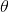虽然未知，但最起码是一个确定的值。

比如如果问那时的人们一个问题：“有一个袋子，里面装着若干个白球和黑球，请问从袋子中取得白球的概率是多少？”他们会立马告诉你，取出白球的概率就是1/2，要么取到白球，要么取不到白球，即θ只能有一个值，而且不论你取了多少次，取得白球的概率θ始终都是1/2，即不随观察结果X 的变化而变化。

这种频率派的观点长期统治着人们的观念，直到后来一个名叫Thomas Bayes的人物出现。

频率派把需要推断的参数θ看做是固定的未知常数，即概率虽然是未知的，但最起码是确定的一个值，同时，样本X 是随机的，所以频率派重点研究样本空间，大部分的概率计算都是针对样本X 的分布；

最大似然估计(MLE)和最大后验估计(MAP)都是把待估计的参数看作一个拥有固定值的变量，只是取值未知。通常估计的方法都是找使得相应的函数最大时的参数；由于MAP相比于MLE会考虑先验分布的影响，所以MAP也会有超参数，它的超参数代表的是一种信念(belief)，会影响推断(inference)的结果。比如说抛硬币，如果我先假设是公平的硬币，这也是一种归纳偏置(bias)，那么最终推断的结果会受我们预先假设的影响。

## 贝叶斯思想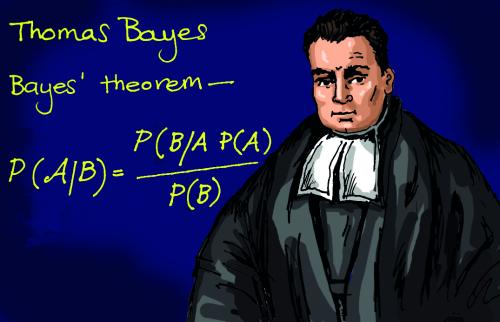回到上面的例子：“有一个袋子，里面装着若干个白球和黑球，请问从袋子中取得白球的概率θ是多少？”贝叶斯认为取得白球的概率是个不确定的值，因为其中含有机遇的成分。比如，一个朋友创业，你明明知道创业的结果就两种，即要么成功要么失败，但你依然会忍不住去估计他创业成功的几率有多大？你如果对他为人比较了解，而且有方法、思路清晰、有毅力、且能团结周围的人，你会不由自主的估计他创业成功的几率可能在80%以上。这种不同于最开始的“非黑即白、非0即1”的思考方式，便是贝叶斯式的思考方式。

贝叶斯派既然把看做是一个随机变量，所以要计算的分布，便得事先知道的无条件分布，即在有样本之前（或观察到X之前），有着怎样的分布呢？

比如往台球桌上扔一个球，这个球落会落在何处呢？如果是不偏不倚的把球抛出去，那么此球落在台球桌上的任一位置都有着相同的机会，即球落在台球桌上某一位置的概率服从均匀分布。这种在实验之前定下的属于基本前提性质的分布称为先验分布，或的无条件分布。

贝叶斯派认为待估计的参数是随机变量，服从一定的分布，而样本X 是固定的，由于样本是固定的，所以他们重点研究的是参数的分布。

### 贝叶斯及贝叶斯派思考问题的固定模式

先验分布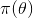+ 样本信息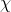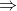后验分布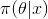上述思考模式意味着，新观察到的样本信息将修正人们以前对事物的认知。换言之，在得到新的样本信息之前，人们对的认知是先验分布，在得到新的样本信息后，人们对的认知为其中，先验信息一般来源于经验跟历史资料。比如林丹跟某选手对决，解说一般会根据林丹历次比赛的成绩对此次比赛的胜负做个大致的判断。再比如，某工厂每天都要对产品进行质检，以评估产品的不合格率θ，经过一段时间后便会积累大量的历史资料，这些历史资料便是先验知识，有了这些先验知识，便在决定对一个产品是否需要每天质检时便有了依据，如果以往的历史资料显示，某产品的不合格率只有0.01%，便可视为信得过产品或免检产品，只每月抽检一两次，从而省去大量的人力物力。

而后验分布一般也认为是在给定样本的情况下的条件分布，而使达到最大的值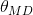称为最大后验估计。

皮皮blog

# 贝叶斯定理

条件概率

条件概率（又称后验概率）就是事件A在另外一个事件B已经发生条件下的发生概率。条件概率表示为P(A|B)，读作“在B条件下A的概率”。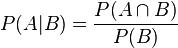联合概率表示两个事件共同发生的概率。A与B的联合概率表示为或者边缘概率（又称先验概率）是某个事件发生的概率。边缘概率是这样得到的：在联合概率中，把最终结果中那些不需要的事件通过合并成它们的全概率，而消去它们（对离散随机变量用求和得全概率，对连续随机变量用积分得全概率），这称为边缘化（marginalization），比如A的边缘概率表示为P(A)，B的边缘概率表示为P(B)。

## 贝叶斯定理

贝叶斯定理是关于随机事件A和B的条件概率和边缘概率的一则定理。在参数估计中可以写成下面这样：

这个公式也称为逆概率公式，可以将后验概率转化为基于似然函数和先验概率的计算表达式，即

在贝叶斯定理中，每个名词都有约定俗成的名称：

P(A)是A的先验概率或 边缘概率。之所以称为"先验"是因为它不考虑任何B方面的因素。
P(A|B)是已知B发生后A的 条件概率(在B发生的情况下A发生的可能性)，也由于得自B的取值而被称作 A的后验概率
P(B|A)是已知A发生后B的条件概率，也由于得自A的取值而被称作B的后验概率。
P(B)是B的先验概率或边缘概率，也作 标准化常量（normalized constant）.

按这些术语，Bayes定理可表述为：

后验概率 = (相似度*先验概率)/标准化常量，也就是说，后验概率与先验概率和相似度的乘积成正比。

另外，比例P(B|A)/P(B)也有时被称作标准相似度（standardised likelihood），Bayes定理可表述为：

后验概率 = 标准相似度*先验概率

## 贝叶斯估计的例子

假设中国的大学只有两种：理工科和文科，这两种学校数量的比例是1:1，其中，理工科男女比例7:1，文科男女比例1:7。某天你被外星人随机扔到一个校园，问你该学校可能的男女比例是多少？然后，你实际到该校园里逛了一圈，看到的5个人全是男的，这时候再次问你这个校园的男女比例是多少？

1. 因为刚开始时，有先验知识，所以该学校的男女比例要么是7:1，要么是1:7，即P(比例为7:1) = 1/2，P(比例为1:7) = 1/2。
2. 然后看到5个男生后重新估计男女比例，其实就是求P(比例7:1|5个男生）= ？，P(比例1:7|5个男生) = ？
3. 用贝叶斯公式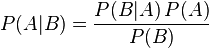，可得：P(比例7:1|5个男生) = P(比例7:1)*P(5个男生|比例7:1) / P(5个男生)，P(5个男生)是5个男生的先验概率，与学校无关，所以是个常数；类似的，P(比例1:7|5个男生) = P((比例1:7)*P(5个男生|比例1:7)/P(5个男生)。
4. 最后将上述两个等式比一下，可得：P(比例7:1|5个男生)/P(比例1:7|5个男生) = {P((比例7:1)*P(5个男生|比例7:1)} / { P(比例1:7)*P(5个男生|比例1:7)}。

## 频率派与贝叶斯派的区别

频率派把需要推断的参数θ看做是固定的未知常数，即概率虽然是未知的，但最起码是确定的一个值，同时，样本X 是随机的，所以频率派重点研究样本空间，大部分的概率计算都是针对样本X 的分布；
贝叶斯派的观点则截然相反，他们认为参数是随机变量，而样本X 是固定的，由于样本是固定的，所以他们重点研究的是参数的分布。

### 两者的本质区别

根据贝叶斯法则：

                posterior=likelihood⋅priorevidence 

                p(ϑ|X)=p(X|ϑ)⋅p(ϑ)p(X) 

在MLE和MAP中，由于是要求函数最大值时的参数，所以都不会考虑evidence。但在贝叶斯估计中，不再直接取极值，所以还会考虑evidence，下面的这个积分也是通常贝叶斯估计中最难处理的部分： 

                p(X)=∫ϑ∈Θp(X|ϑ)p(ϑ)dϑ 

evidence相当于对所有的似然概率积分或求和(离散时)，所以也称作边界似然

### 估计未知参数所采用的思想不同的例子

我去一朋友家：

按照频率派的思想，我估计他在家的概率是1/2，不在家的概率也是1/2，是个定值。

按照贝叶斯派的思想，他在家不在家的概率不再认为是个定值1/2，而是随机变量。比如按照我们的经验（比如当天周末），猜测他在家的概率是0.6，但这个0.6不是说就是完全确定的，也有可能是0.7。如此，贝叶斯派没法确切给出参数的确定值（0.3,0.4，0.6,0.7，0.8,0.9都有可能），但至少明白哪些取值（0.6,0.7，0.8,0.9）更有可能，哪些取值（0.3,0.4） 不太可能。进一步，贝叶斯估计中，参数的多个估计值服从一定的先验分布，而后根据实践获得的数据（例如周末不断跑他家），不断修正之前的参数估计，从先验分布慢慢过渡到后验分布。

各种参数估计方法可以参考Heinrich论文的第二部分。

[[各种参数估计方法的论述：Gregor Heinrich.Parameter estimation for text analysis*]

数理统计学简史》

《统计决策论及贝叶斯分析 James O.Berger著》

[ 机器学习之用Python从零实现贝叶斯分类器]]

皮皮blog

# 贝叶斯估计

贝叶斯估计是在MAP上做进一步拓展，此时不直接估计参数的值，而是允许参数服从一定概率分布。极大似然估计和极大后验概率估计，都求出了参数theta的值，而贝叶斯推断则不是，贝叶斯推断扩展了极大后验概率估计MAP（一个是等于，一个是约等于）方法，它根据参数的先验分布P(theta)和一系列观察X，求出参数theta的后验分布P(theta|X)，然后求出theta的期望值，作为其最终值。另外还定义了参数的一个方差量，来评估参数估计的准确程度或者置信度。

贝叶斯公式

现在不是要求后验概率最大，这样就需要求,即观察到的evidence的概率，由全概率公式展开可得

当新的数据被观察到时，后验概率可以自动随之调整。但是通常这个全概率的求法是贝叶斯估计比较有技巧性的地方。

### 用贝叶斯估计来做预测

如果我们想求一个新值的概率，可以由下面公式来计算。

此时第二项因子在上的积分不再等于1，这就是和MLE及MAP很大的不同点。

### 扔硬币的伯努利实验示例

跟上面极大后验概率例子一样，N次伯努利实验，参数p（即正面的概率）的先验分布是参数为（5,5）的beta分布，然后接下来，我们根据参数p的先验分布和N次伯努利实验结果来求p的后验分布。我们假设先验分布为Beta分布，但是构造贝叶斯估计时，不是要求用后验最大时的参数来近似作为参数值，而是求满足Beta分布的参数p的期望，也就是直接写出参数的分布再来求分布的期望，有Note:

1 C是所有实验结果的集合Ci=1或者0。

2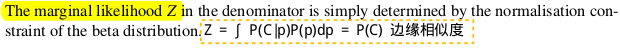3 这里用到了公式

4 推导也可参考[ 主题模型TopicModel：LDA中的数学模型：Beta-Binomial 共轭部分]

根据结果可以知道，根据贝叶斯估计，参数p服从一个新的Beta分布。回忆一下，我们为p选取的先验分布是Beta分布，然后以p为参数的二项分布用贝叶斯估计得到的后验概率仍然服从Beta分布，由此我们说二项分布和Beta分布是共轭分布。当T为二维的情形可以对Beta分布来应用；T为多维的情形可以对狄利克雷分布应用。

根据Beta分布的期望和方差计算公式，我们有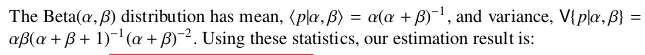可以看出此时估计的p的期望和MLE ，MAP中得到的估计值都不同，此时如果仍然是做20次实验，12次正面，8次反面，那么我们根据贝叶斯估计得到的p满足参数为12+5和8+5的Beta分布，其均值和方差分别是17/30=0.567, 17*13/(31*30^2)=0.0079。可以看到此时求出的p的期望比MLE和MAP得到的估计值都小，更加接近0.5。

皮皮blog

# 贝叶斯参数估计

贝叶斯方法中，把参数视为影响所有训练实例概率的未观测变量。于是学习相当于根据观测来计算新样本的概率，这种学习可以通过计算参数上的后验概率（分母也要计算出来？）来执行，且使用它进行预测。

而MAP后验估计是根据最大的p(theta | X)来计算新样本的概率？

### 图钉和硬币示例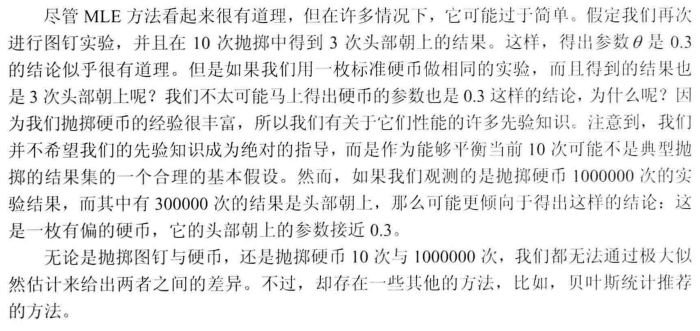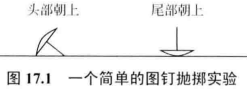## 联合概率模型

将贝叶斯学习视为包含所有实例和参数中所有变量的meta-网中的推理问题。计算未来事件的概率相当于给定前M个观测实例时，关于第M+1个实例的后验概率执行查询。

用一个概率分布来表示参数theta的先验知识，在theta和观测的数据X上建立一个联合分布。（也就是将theta也当成一个随机变量，而不是一个待估参数）

theta固定时，不同抛掷间的条件独立；theta未知时，每次抛掷都可以传递一些有关参数theta的信息，不同抛掷间的边缘独立性不成立。这种直观和贝叶斯网的独立性是一致的！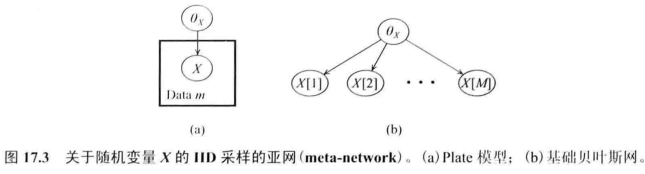Note：X的每次取值代表每次抛掷的不同结果。

### 参数和数据的联合分布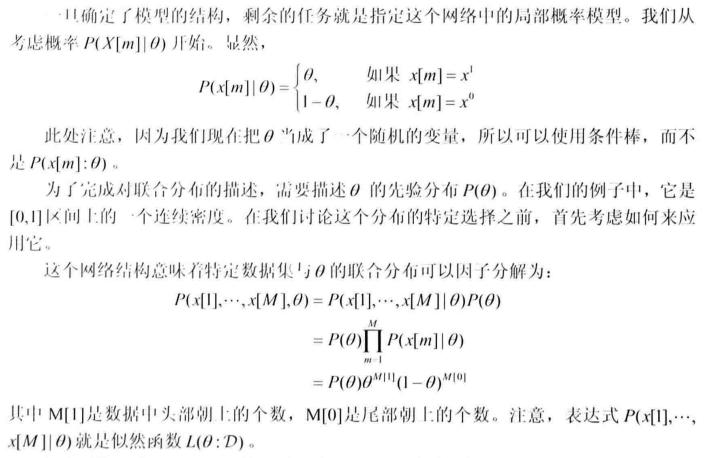Note: 公式与MLE唯一的区别只在于多了一个P(theta)。也要注意其和贝叶斯网的MLE估计的区别[]。

### 联合分布、后验分布和似然、先验的联系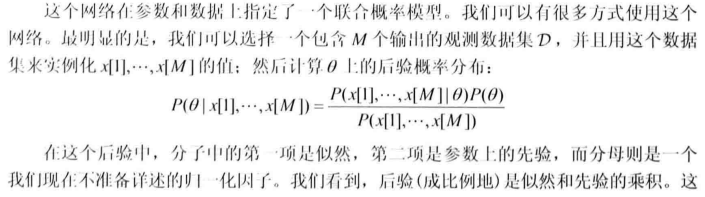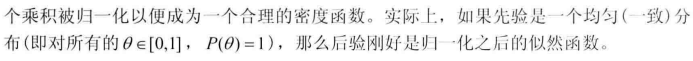## 预测：贝叶斯估计子预测和拉普拉斯校正

给定前M次抛掷的结果来推理第M+1次抛掷的值。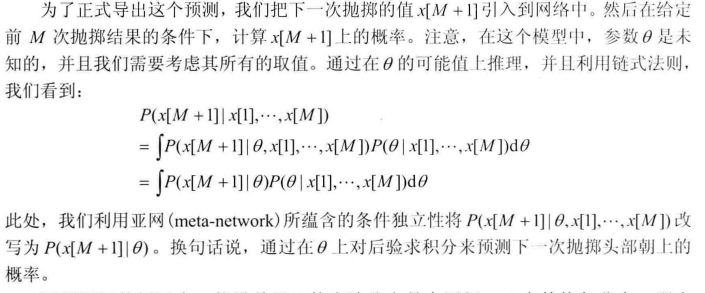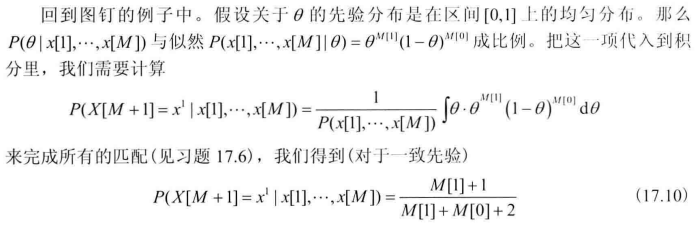Note: 公式推导中P(x[M+1] = x1 | theta) 就是theta； P(x...)仅是一个归一化因子，不用直接计算，分别计算X[]=x1和x0再相加就可以了。

贝叶斯估计子预测公式推导：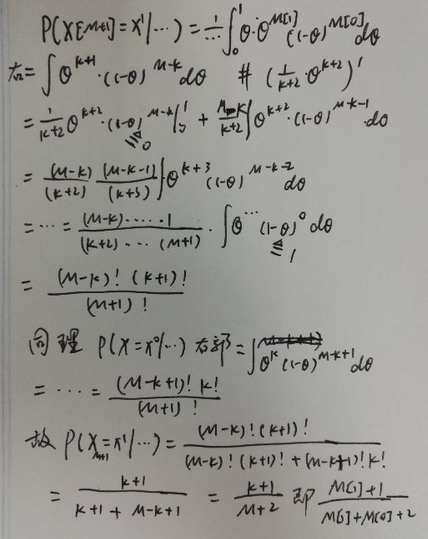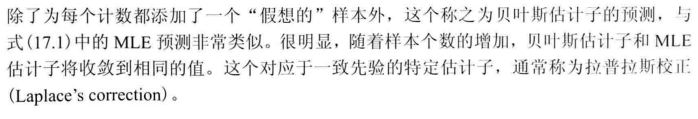## 先验

非均匀的先验分布

### 选择beta分布作为伯努利分布的先验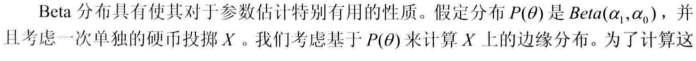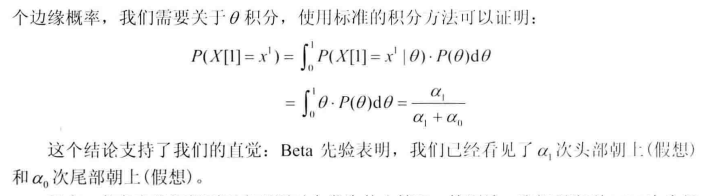### 共轭性质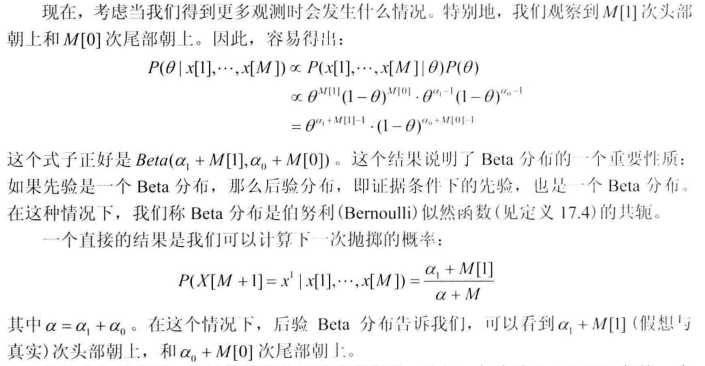### 先验强度与样本量：先验分布对参数估计的影响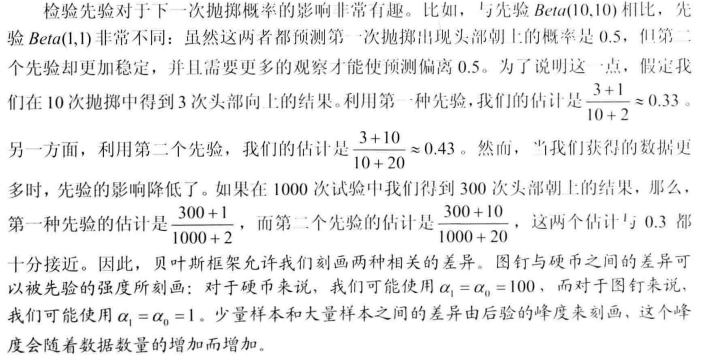## 先验分布与后验分布

### 参数和数据的联合分布### 先验和后验的形式

对某些概率模型，似然函数可以用充分统计量紧凑表示。而这里，后验分布同样也可以紧凑表示，而这就取决于先验的形式。如先验分布为beta分布，那么二项式分布的数据的后验分布也是紧凑的beta分布；先验分布为dirichlet分布，那么多项式分布的数据的后验分布也是紧凑的dirichlet分布。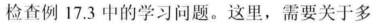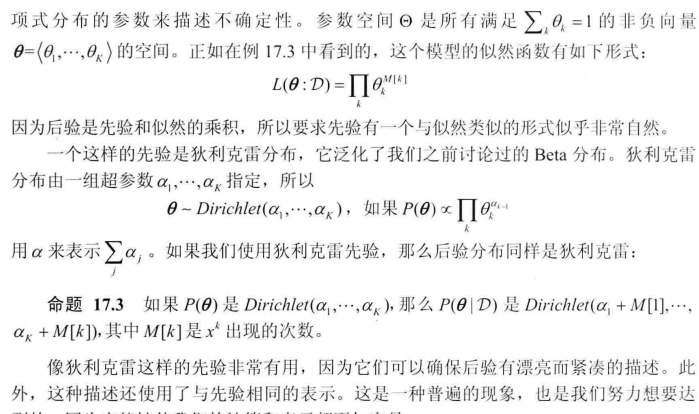### 共轭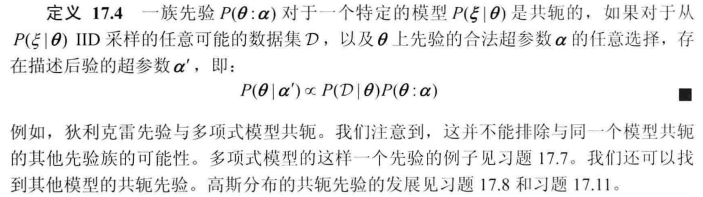### 后验概率分布的作用：确定模型属性（如偏置）和预测新数据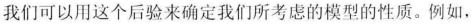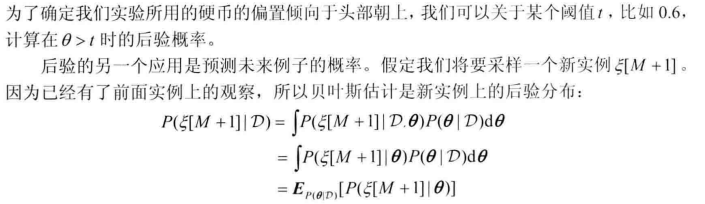### Dirichlet先验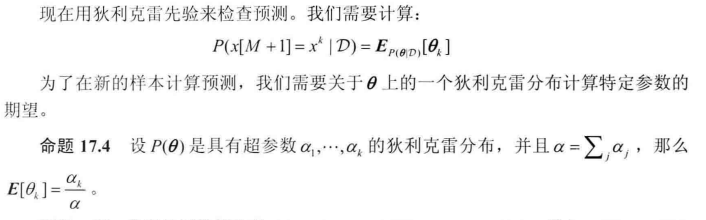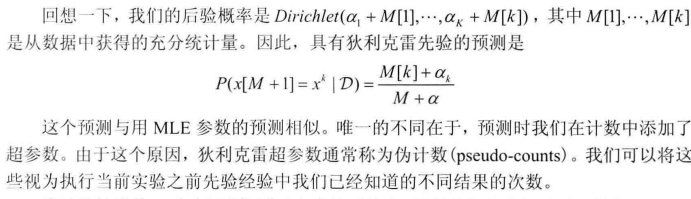### 先验强度alpha和先验均值theta‘

直观上，当我们有一个很大的训练集时，先验的作用是可以忽略不计的。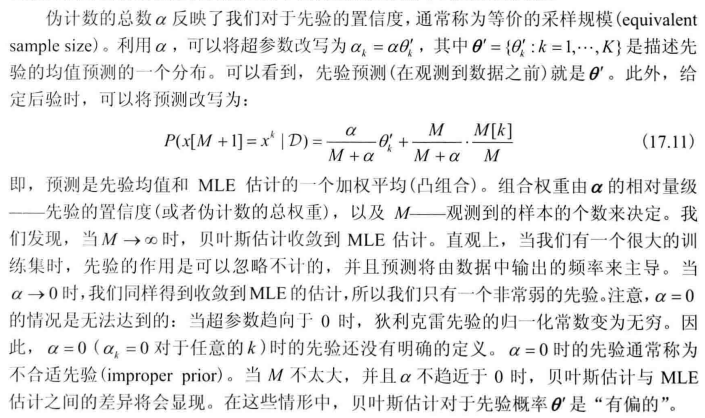先验强度和均值对估计的影响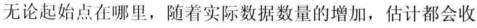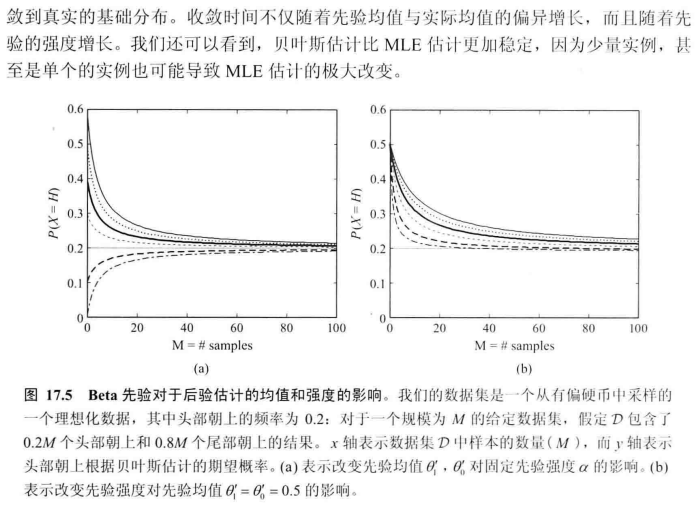可以看到，这种先验起到了平滑的效果，导致了更加鲁棒的估计

Note: 另先验可以避免overfitting，这是因为先验带来的伪计数减小了训练数据的偏倚

先验不能使用极值估计

实际为0的概率的估计是很危险的，因为无论多大量的证据都无法改变它们。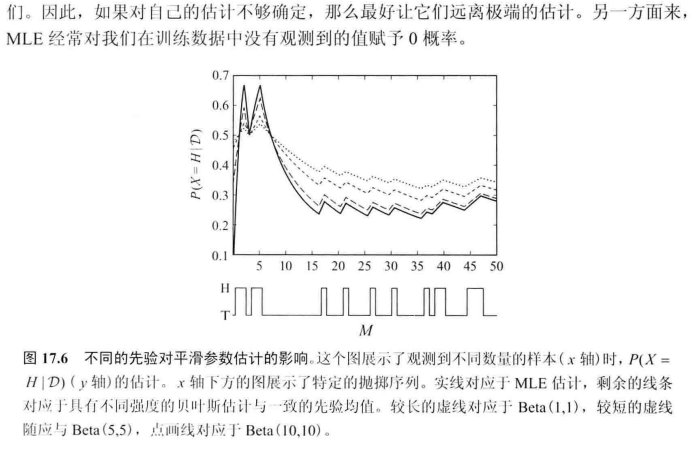[《Probabilistic Graphical Models：Principles and Techniques》(简称PGM)]

ref:  [天真的贝叶斯，神奇的贝叶斯方法]

展开全文• 贝叶斯估计和最大似然估计的原理及代码matlab
• 机器学习笔记—模式分类（二）参数判别估计法（最大似然估计和贝叶斯参数估计）1 机器学习笔记—模式分类（三）参数判别估计法2（最大似然估计） 同类文章： 机器学习笔记—模式识别与智能计算（四）基于概率统计...

前序文章：

机器学习笔记—模式分类（一）绪论&贝叶斯决策论

机器学习笔记—模式分类（二）参数判别估计法（最大似然估计和贝叶斯参数估计）1

机器学习笔记—模式分类（三）参数判别估计法2（最大似然估计）

同类文章：

机器学习笔记—模式识别与智能计算（四）基于概率统计的贝叶斯分类器设计（贝叶斯决策）

3、贝叶斯估计

在最大似然估计方法中，把需要估计的参数向量θ看作是一个确定而未知的参数；而在贝叶斯学习方法中，把参数向量θ看成一个随机变量，已有的训练样本使我们能够把对于θ的初始密度p(θ)的估计转化为后验概率密度p(θ|D)。

贝叶斯估计的假设：

（1）现有的全部信息一部分为我们的先验知识，如未知概率密度函数m的形式、未知参数的取值范围等，另一部分则来自训练样本本身；

（2）同最大似然估计，对于c类样本集，如果i!=j，那么样本集Di中的训练样本对p(x|wj,D)没有任何影响，根据贝叶斯公式p(wi|x,D)可以通过p(x|wi,Di)（即后面的p(x|D)）计算得到；由于能够对每一类别进行处理，因此公式中为了说明各个类别的记号都可以省略，从而简化公式的形式；

（3）虽然具体的概率密度p(x)未知，但假设其参数形式已知，唯一未知的是参数向量θ的值，为表示这个意思我们强调条件概率密度p(x|θ)是完全已知的，只是θ未知。

在上述假设条件下，我们要处理的是c个独立的问题，每一个问题都是如下形式：已知一组样本D，这些样本都是从固定但未知的概率密度函数p(x)中独立抽取的，要求根据这些样本估计p(x|D)，这就是贝叶斯学习的核心问题。

在观察到具体的训练样本之前，我们已有的关于参数向量θ的全部知识可以用θ的先验概率密度p(θ)来体现，根据训练样本的观察和贝叶斯公式，θ的后验概率密度p(θ|D)可以通过θ的先验概率密度p(θ)计算得到，并且我们希望p(θ|D)在θ的真实值附近有非常显著的尖峰。

根据贝叶斯估计的核心公式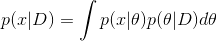类条件概率密度p(x|D)可以通过未知参数量θ的后验概率密度p(θ|D)计算得到。

如果后验密度在某个值θ'附近形成最显著的尖峰，那么有p(x|D)≈p(x|θ')（p(x|θ')可看成是用极大似然估计求出θ'进而求出p(x|θ')的结果），即用估计值θ'近似代替真实值（前提是p(x|θ)光滑且积分拖尾的影响足够小，一般都能满足）。

总结前面的讨论，如果未知的概率密度函数p(x|θ)具有一个已知的形式的话，已有的训练样本D能够通过参数后验密度p(θ|D)对类条件概率密度p(x|D)的估计施加影响。

4、贝叶斯参数估计：高斯情况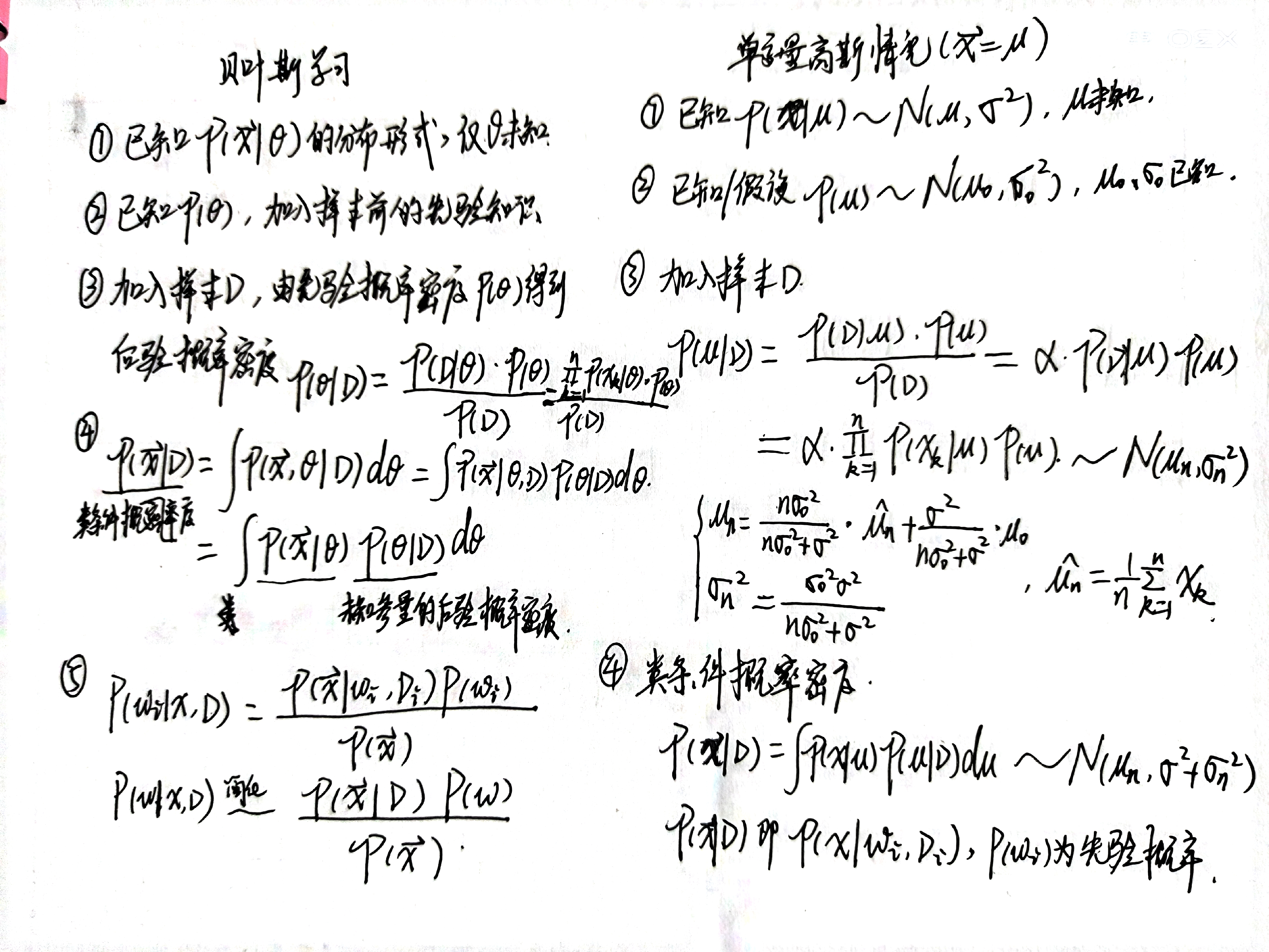对于贝叶斯估计的单变量高斯情况，p(μ|D)的分布参数μn和σn^2公式显示了参数先验知识p(μ)和样本观测结果p(x|μ)是如何被结合在一起并形成参数的后验概率p(μ|D)的；

μn代表了在观察到n个样本后对μ的真实值的最好估计，σn^2反映了对这个估计的不确定程度；

当n增加时，p(μ|D)的波形变得越来越尖，且在n趋于无穷大时，逼近狄拉克函数，这一现象被称为贝叶斯学习过程

为了得到类条件概率密度函数p(x|D)，其参数形式为已知的p(x|μ)服从N(μ, σ^2)，只需用μn替换μ、用 σ^2+σn^2替换σ^2即可；

p(x|D)就是类条件概率密度函数p(x|wi,Di)，结合先验概率P(wi)，我们就完全掌握了设计贝叶斯分类器所需的概率知识。

在这点上贝叶斯估计方法与最大似然方法不同，因为最大似然方法仅仅是估计μ和σ^2的值，而不是估计p(x|D)的分布。

5 贝叶斯参数估计：一般理论

参数估计的递归贝叶斯方法是我们遇到的第一个“增量学习”或在线学习算法，其特点是学习过程随着观察数据的不断获得而不断进行，如果这一概率密度函数的序列最终能够收敛到一个中心在参数的真实值附近的狄拉克函数，那么就实现了贝叶斯学习过程。

与最大似然法不同的是，最大似然法估计的是θ空间中的一个点，而贝叶斯方法估计的则是一个概率分布；严格来说，不能直接比较这两种方法技术本身，只有在计算概率密度p(x|D)时才可能进行一个公平的比较。

对于通常能遇到的典型条件概率密度函数p(x|θ)，未知参量θ后验概率密度函数序列p(θ|Dn)一般都能收敛到狄拉克函数，这就意味着只要训练样本的数量足够多，就能够确定唯一的一个最适合这些训练样本的θ的值，即未知参量θ能够被条件概率密度函数p(x|θ)唯一确定，在这种情况下概率密度函数p(x|θ)被称为可辨识的。

对于参数的先验概率保证问题有解的情况下，最大似然估计和贝叶斯估计在训练样本趋近于无穷时效果是一样的。

在实际训练样本有限的情况下，选取最大似然估计还是贝叶斯估计通常需要考虑几项标准：

（1）计算的复杂度—最大似然法涉及一些微分运算或梯度搜索技术，而贝叶斯估计方法可能要求计算非常复杂的多重积分；

（2）可理解性—最大似然法得到的结果是基于训练样本的一个最佳解答，较容易理解，而贝叶斯估计方法得到的结果是许多可行解答的加权平均值，反映出对各种可行解答的不确定程度，反映出对所使用的模型的剩余的不确定性；

（3）对初始先验知识的信任程度，如对条件概率密度函数p(x|θ)的形式—最大似然估计得到的结果与初始假设的形式一致，而贝叶斯估计未必，通过使用全部p(θ|D)中的信息产生更多有用的信息。在参数没有特别的先验知识的情况下（如均匀分布），贝叶斯估计方法与最大似然估计方法相似，如果有非常多的训练样本，使得p(θ|D)形成一个非常显著的尖峰，而参数先验概率p(θ)又是均匀分布情况下，前面所述的最大后验概率估计（MAP估计）在本质上也是与最大似然估计相同的。

当使用最大似然估计或贝叶斯估计的结果设计分类器时，采用的还是贝叶斯决策论的方法：对每一类别都计算后验概率密度函数p(wi|x)，根据最大后验概率P(wi|x)对测试样本进行分类。那么分类器产生的最终分类误差来源有：

（1）贝叶斯误差或不可分性误差，由于不同类别的类条件概率密度p(x|wi)之间的画像重叠引起的，这种分类误差时问题本身所固有的，因此永远无法消除；

（2）模型误差，由于选择了不正确的模型所导致的分类误差，这一误差在最大似然和贝叶斯估计中的影响时类似的；

（3）估计误差，由于采用有限样本进行估计带来的误差。在理论上贝叶斯估计有很强的理论和算法基础；但在实际应用中，最大似然估计更加简便，设计出的分类器性能几乎与贝叶斯方法得到的结果相差无几。

无信息先验：在贝叶斯决策论中，在处理每一类别的先验概率时，如果没有其他的特别信息，那么我们都简单地建设每一类的概率相同；类似地，在贝叶斯估计方法中，我们对每一个参数也有一个无信息的先验估计，如满足平移不变性等。

如果已经知道必须满足的不变性如平移不变性、对离散分布要求样本选取顺序的无关性，那么就会对先验概率的可能具有的形式带进约束，如果能找到满足这种约束的分布，那么最后的结果就称为对这些不变性要求是无信息的。

在前述假设下，贝叶斯最优分类器能够达到最佳的分类效果，然而类条件概率密度的积分可能非常复杂，为减低难度，一个变通的方法是依据参数后验密度p(θ|D)仅仅选取一个参数向量θ并且把它当做真实值，这就是吉布斯/Gibbs算法，在较弱的条件下，Gibbs算法的误差概率至多是贝叶斯最优分类器的两倍。

展开全文机器学习 python 人工智能
• 基于贝叶斯参数估计和扩展有限元的结构损伤识别研究，严刚，，本文提出了一种基于贝叶斯参数估计和扩展有限元的方法，对结构中损伤的位置和尺寸进行识别。该方法在获得测量信息后，结合结构损
• 相对论重离子碰撞的贝叶斯参数估计码 请使用提供的environment.yml生成兼容的conda环境。 有关更多信息，请参阅 conda env创建--file environment.yml 获取实验数据文件夹并解压缩模拟数据 sh prepare.sh
• 山东大学计算机科学与技术学院机器学习课程的实验报告
• 贝叶斯模型选择程序使用贝叶斯统计方法来评估三个模型中的哪一个最能代表示例数据。 会在10小时，24小时和100小时内“收集”数据，以便我们评估随着更多数据的引入，我们的模型选择可能会如何变化。 绘制了一些示例...Python
• 直接统计类条件密度太复杂了且样本不足，所以我们希望用一个密度函数去拟合它，比如拟合成下面的正态分布，其中的参数可以用最大似然方法或者贝叶斯估计去进行参数估计。 给每个类建模一个密度函数，每个类的函数...机器学习 深度学习
• 　参数估计是统计学中的经典问题，常用的方法是最大似然估计和贝叶斯估计。为什么机器学习中，也会用到参数估计呢？我们利用训练样本来估计先验概率和条件概率密度，并以此设计分类器。当假设数据符合某种分布时，其...机器学习 模式识别
• 贝叶斯分类器估计后验概率的问题可转换为估计类先验概率和类条件概率。对于朴素贝叶斯，假设属性条件性独立，因此核心就是估计类先验概率和各属性的类条件概率。尽管朴素贝叶斯分类器的假设过于简化，但是有些实际...机器学习
• 贝叶斯估计的算法过程合极大似然估计的算法过程一模一样，代码也几乎一模一样，只是加了一个λ。 2. Python代码 def priorProbability ( labelList , Lambda ) : # 加入一个lambda labelSet = set ( ...
• 3.3 贝叶斯估计估计和极大似然估计方法的优点是比较客观客观，基本由随机采样数据决定。缺点是需要在大样本情况下估计才比较准确。不能把人类知识用于估计。例如，某公司研发新产品，需要估计合格率，这是典型的...机器学习
• 方法有最大似然估计和贝叶斯参数估计法。 最大似然估计 假设参数为确定值，根据似然度最大进行最优估计。 给定数据 D 1 , D 2 . . . D c D_1,D_2...D_c D 1 ​ , D 2 ​ . . . D c ​ 表示不同类别的样本。...
• 贝叶斯统计学是一门基本思想与传统基于频率思想的统计学完全不同的统计学方法；它以其灵活 性和先进性在现代的统计学中占据着重要的地位。...1.3 稀少事件的概率估计 1.4 可交换性 1.5 预测模型的构建 单参数模型 2傅立叶分析
• 最大似然估计与贝叶斯参数估计 前言 上一篇讲到了贝叶斯决策理论，其与贝叶斯估计是两种不同的思想。前者是根据先验概率P(ωi)P(ωi)P(\omega_i)和类条件概率密度p(x|ωi)p(x|ωi)p(x|\omega_i)来设计最优分类器...
• 1/11/2017 11:02:08 PM 考试结束了重新看了一下贝叶斯参数估计（极大似然参数估计思想很简单，不用多说了），感觉贝叶斯参数估计真是内涵很深啊！ 下面两张ppt务必完全每一行都看懂（刘老师的PPT确实做得好啊） 第...
• 即随着样本量的增加，估计量的值收敛（概率）为基础参数的真实值，即期望值估计量可能与真实值有所不同。 下面的例子给出了一个有偏估计。假设我们参加一次抽奖，从一个抽奖盒中抽出不同颜色的球，一次一个。我们...机器学习 数据挖掘 最大似然
• 贝叶斯判决下的最大似然估计与贝叶斯估计 描述了两种参数估计的具体方法和区别最大似然估计 参数估计 机器学习
• 贝叶斯学派认为，除了上述两种信息以外，统计推断还应该使用第三种信息：先验信息。下面我们先把是那种信息加以说明。 总体信息：总体信息即总体分布或总体所属分布族提供的信息。譬如，若已知“总体是正态分布”......